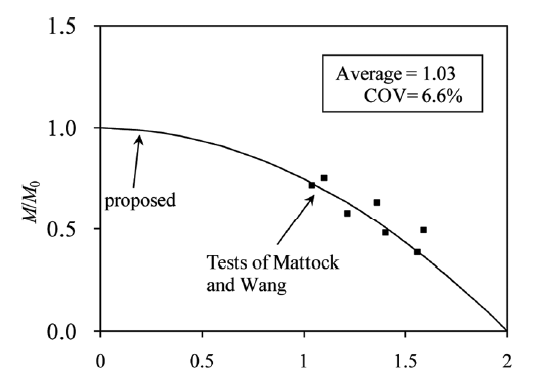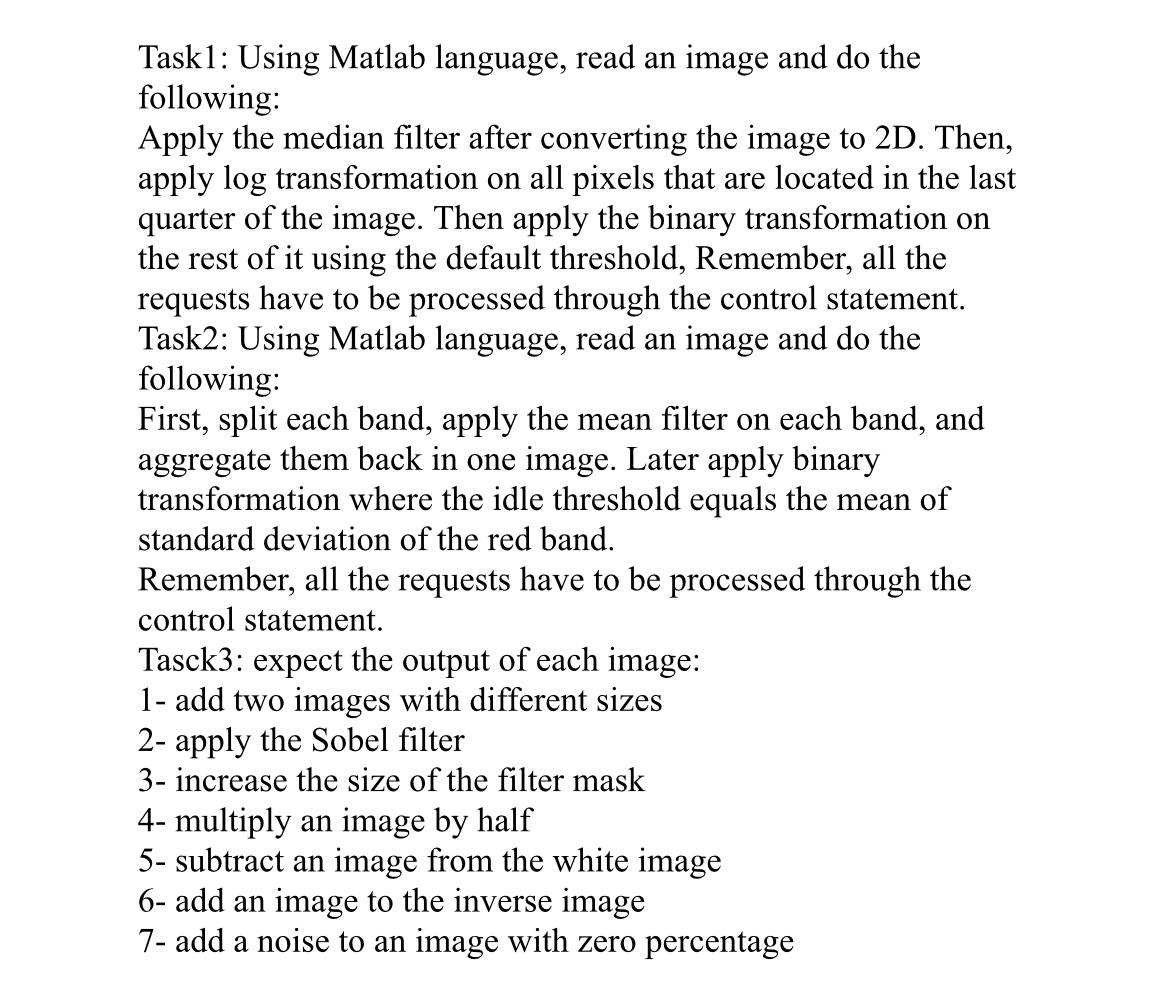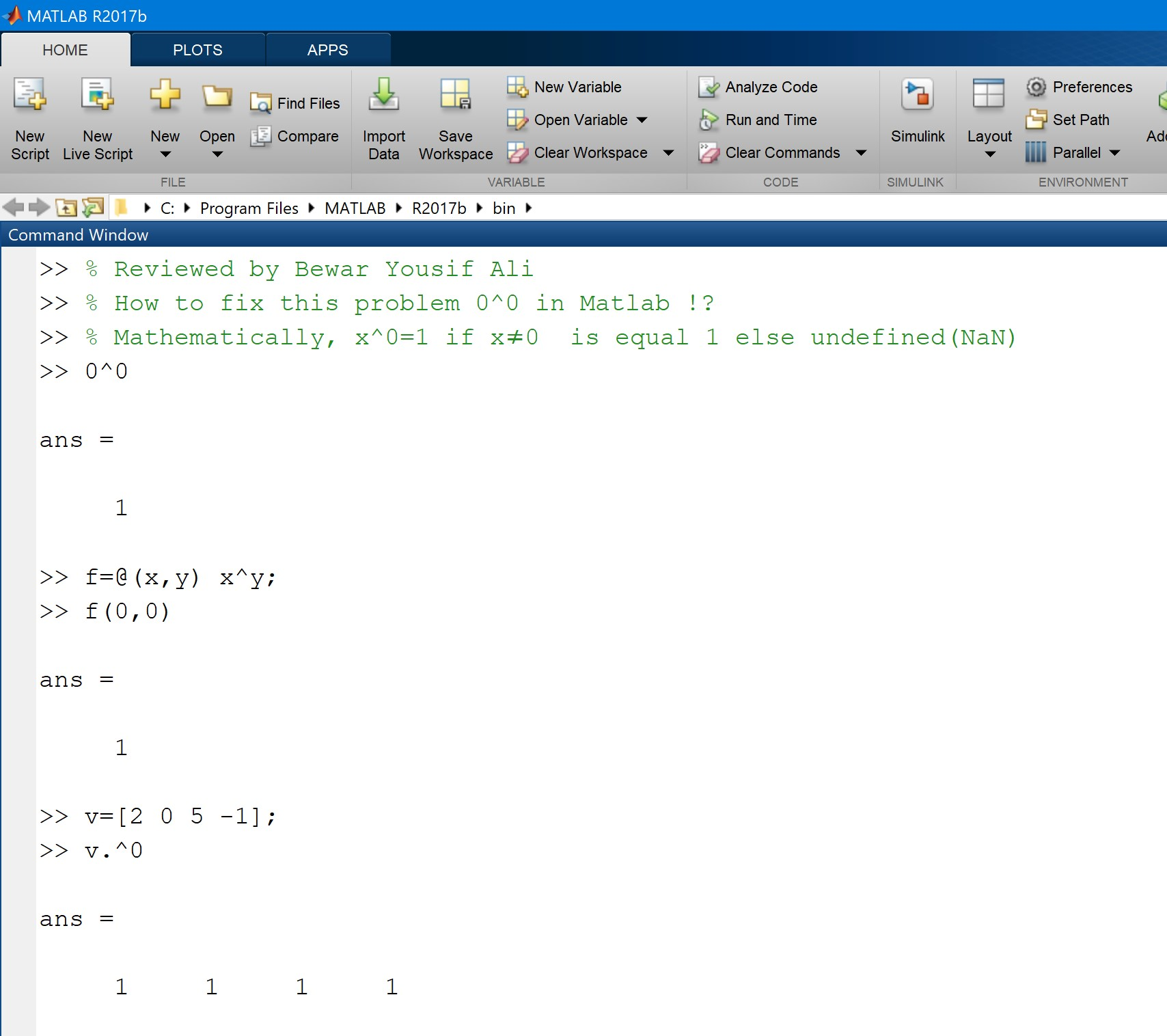Pull up a chair!

Discussions is your place to get to know your peers, tackle the bigger challenges together, and have fun along the way.

• Looking for techniques improve your MATLAB or Simulink skills? Tips & Tricks has you covered!
• Sharing the perfect math joke, pun, or meme? Look no further than Fun!
• Think there's a channel we need? Tell us more in Ideas

Kittiwith
Posted etwa 15 Stunden vor

### Error using plannerBiRRT/plan

This is my code
%send food and medicine in flood situation
% 1. create map
clc
clear
tic
map3D = occupancyMap3D; % create space for
[xGround,yGround,zGround] = meshgrid(0:240,0:350, 0); %set floor x=500 y=500
xyzGround = [xGround(:) yGround(:) zGround(:)]; %use for mergegrid
occval = 0;
setOccupancy(map3D,xyzGround,occval)
% xspace = 20 y = 45 create
[xB1, yB1, zB1] = meshgrid(50:130, 60:110, 0:20); %unionmall
[xB2, yB2, zB2] = meshgrid(80:250, 160:240, 0:30); %centralladprao
[xB3, yB3, zB3] = meshgrid(220:250, 290:340, 0:60); %centarahotel
[xB4, yB4, zB4] = meshgrid(0:30, 10:40, 0:80); %condo
[xB5, yB5, zB5] = meshgrid(150:170, 80:100, 0:10); %house1
[xB6, yB6, zB6] = meshgrid(180:200, 80:100, 0:10); %house2
[xB7, yB7, zB7] = meshgrid(210:230, 80:100, 0:10); %house3
[xB11, yB11, zB11] = meshgrid(240:270, 80:100, 0:10); %house7
[xB8, yB8, zB8] = meshgrid(150:170, 50:70, 0:10); %house4
[xB9, yB9, zB9] = meshgrid(180:200, 50:70, 0:10); %house5
[xB10, yB10, zB10] = meshgrid(210:230, 50:70, 0:10); %house6
[xB12, yB12, zB12] = meshgrid(240:270, 50:70, 0:10); %house8
[xB13, yB13, zB13] = meshgrid(200:260, 0:30, 0:13); %villageoffice
xyzB = [xB1(:), yB1(:), zB1(:);
xB2(:), yB2(:), zB2(:);
xB3(:), yB3(:), zB3(:);
xB4(:), yB4(:), zB4(:);
xB5(:), yB5(:), zB5(:);
xB6(:), yB6(:), zB6(:);
xB7(:), yB7(:), zB7(:);
xB8(:), yB8(:), zB8(:);
xB9(:), yB9(:), zB9(:);
xB10(:), yB10(:), zB10(:);
xB11(:), yB11(:), zB11(:);
xB12(:), yB12(:), zB12(:);
xB13(:), yB13(:), zB13(:);];
obs = 0.65; % ;=not show in command window
my3Dmap = map3D;
updateOccupancy(my3Dmap,xyzB,obs);
%show(my3Dmap);
% 2. distination
%my3Dmap.FreeThreshold = my3Dmap.OccupiedThreshold;
startPose = [50 60 25 pi/2;
250 310 65 pi/2;
100 170 35 pi/2;
100 30 85 pi/2;
155 90 15 pi/2;
250 60 15 pi/2;
160 60 15 pi/2
220 15 20 pi/2;];
goalPose = startPose;
ss = ExampleHelperUAVStateSpace("MaxRollAngle",pi/3,...
"AirSpeed",5,...
"FlightPathAngleLimit",[-0.5 0.5],...
"Bounds",[-10 400;-10 4000; -10 100; -pi pi]);
%threshold = [(goalPose-0.5)' (goalPose+0.5)'; -pi pi];
%setWorkspaceGoalRegion(ss,goalPose,threshold)
sv = validatorOccupancyMap3D(ss,"Map",my3Dmap);
sv.ValidationDistance = 0.5;
planner = plannerBiRRT(ss,sv);
planner.MaxConnectionDistance = 100;
%planner.GoalBias = 0.10;
%1,0.1,0.15,0.5,0.2,0.13,
planner.MaxIterations = 100000;
%planner.GoalReachedFcn = @(~,x,y)(norm(x(1:3)-y(1:3)) < 5);
planner.MaxNumTreeNodes = 10000;
numPose = length(startPose);
distances = zeros(numPose, numPose);
for i = 1:numPose
for j = 1:numPose
if i ~= j
rng(100, "twister");
start = [startPose(i,1), startPose(i,2), startPose(i,3), startPose(i,4)];
goal = [goalPose(j,1), goalPose(j,2), goalPose(j,3), goalPose(j,4)];
[pthObj, solnInfo] = plan(planner, start, goal);
smoothWaypointsObj = exampleHelperUAVPathSmoothing(ss, sv, pthObj);
distances(i, j) = pathLength(smoothWaypointsObj);
end
end
end
toc
and the error belowGumaa Abdelrhim
Posted am 10 Dez. 2023 um 20:19

### Moment- axial force intrectionHi, I have this equation for moment-axial force interaction: 0.25*(M/M0)^2 + (N/N0) = 1. This equation produces the following curve. Now, I need to make a change to this equation so that the first term becomes (M/M0)^2, and the second term can be changed while keeping (N/N0). The other side of the equation remains the same. ((M/M0)^2+?? = 1). I need to get the same curve as in the figure.
Yoann
Posted am 10 Dez. 2023 um 18:49

### ultrasound video clip analysis

...Ghg
Posted am 9 Dez. 2023 um 14:29

### I need solutions for this three tasksJoseph
Posted am 8 Dez. 2023 um 16:24

### solved

Solved
Riccardo Spartà
Posted am 8 Dez. 2023 um 10:36

### How to get real-time outputs and real-time inputs from/to Simulink, without using Simulink Real-Time?

Hi everyone,
for my thesis project I would need to get, every second of the simulation, real-time output data from a Simulink model, without using Simulink Real-Time, as it supports only Speedgoat Hardware. Is there a particular set of blocks for that purpose?
I have to provide that real-time data to hardware for an Hardware in the Loop Simulation so I will need to be able also to receive, as an input, data from the hardware to the Simulink model, again every second of the simulation, to close the loop of the simulation.
Sushil Kunwar
Posted am 8 Dez. 2023 um 0:02

### Curve line plot

Hi! I am using matlab inbuilt plot option( without typing code) to plot the data. This joins the data points using straight line by default. What will be the easiest way to plot if I want curve to join the points instead?
Jan Studnicka
Posted am 7 Dez. 2023 um 12:06

### How to make corrplot from Econometrics Toolbox 2x faster?

Explanation: Function corrplot uses functions plotmatrix and lsline. In lsline get(hh(k),'Color') is called in for cycle for each line and scatter object in axes. Inside the corrplot it is also called for all axes, which is slow. However, when you first set the color to any given value, internal optimization makes it much faster. I chose [0 0.4470 0.7410], because it is a default color for plotmatrix and corrplot and this setting doesn't change a behavior of corrplot.
Suggestion for a better solution: Add the line of code set(hS,'Color',[0 0.4470 0.7410]) to the function plotmatrix. This will make not only corrplot faster, but also any other possible combinations of plotmatrix and get functions called like this:
h = plotmatrix(A);
% set(h,'Color',[0 0.4470 0.7410])
for k = 1:length(h(:))
get(h(k),'Color');
end
Hans Scharler
Posted am 4 Dez. 2023 um 19:29

### New Community Feature: AI Chat Playground

The MATLAB AI Chat Playground is now open to the whole community! Answer questions, write first draft MATLAB code, and generate examples of common functions with natural language.
The playground features a chat panel next to a lightweight MATLAB code editor. Use the chat panel to enter natural language prompts to return explanations and code. You can keep chatting with the AI to refine the results or make changes to the output.Give it a try, provide feedback on the output, and check back often as we make improvements to the model and overall experience.
Grace Woolson
Posted am 4 Dez. 2023 um 17:15

Looking for an opportunity to practice your AI skills on a real-world problem? Interested in AI for climage change? Sign up for the Kelp Wanted challenge, which tasks participants with developing an algorithm that can detect the presence of kelp forests from satellite images.
Participants of all skill levels from anywhere in the world are welcome to compete!
MathWorks provides the following resources for all participants:
Siebe Caems
Posted am 30 Nov. 2023 um 16:28

### Difference rotating over moving angle and normal angle

Hello,
What is the difference between a moving angle and a normal angle?
Compute the Rotation about Y-axis over moving angle a = 30°
then
rotation about X-axis of angle β= 45°
What will the code like?
Thank you
Kali
Posted am 30 Nov. 2023 um 5:57

### Help

Write a matlab script that will print the odd numbers, 1 through 20, in reverse.
ABOAJELA
Posted am 29 Nov. 2023 um 15:53

### damping circuit

i need circuti to model wound rotor synchronous machine damping circuit in matlab simulink , is there any one know how to do it
Ayush
Posted am 29 Nov. 2023 um 10:06

### facing an issue in

we have extracted a .xml file from solidworks to import in matlab, when we tried to import it shows that simscape multibody should be installed and when we tried to install it it shows the message attached below.
we are working on an educational license provided by our university, is it the issue?
Bewar Yousif Ali
Posted am 27 Nov. 2023 um 18:08

### How to fix this problem 0^0 in Matlab !?

It is crucial to understand that this expression could be used in problems related to engineering, physics, mathematics, or any other aspect of real life.
Typically, Matlab is used to solve PDE and ODE problems. Perhaps users calculated this term 0^0 incorrectly in the process.>> % Reviewed by Bewar Yousif Ali
>> % How to fix this problem 0^0 in Matlab !?
>> % Mathematically, x^0=1 if x≠0 is equal 1 else undefined(NaN)
>> 0^0
ans =
1
>> f=@(x,y) x^y;
>> f(0,0)
ans =
1
>> v=[2 0 5 -1];
>> v.^0
ans =
1 1 1 1
Chen Lin
Posted am 27 Nov. 2023 um 17:28

### Vote on interesting MATLAB animations and win MATLAB T-shirts!

What amazing animations can be created with no more than 2000 characters of MATLAB code? Check out our GALLERY from the MATLAB Flipbook Mini Hack contest.
Vote on your favorite animations before Dec. 3rd. We will give out MATLAB T-shirts to 10 lucky voters!Tips: the more you vote, the higher your chance to win.
JUAN CARLOS
Posted am 26 Nov. 2023 um 3:27

### i=dsolve('Dy=-8*y+40*sin(8*t)','y(0)=5') Warning: Support for character vector or string inputs will be removed in a future release. Instead, use syms to declare variables and replace inputs such as dsolve('Dy = -3*y') with syms y(t); dsolve(diff(y,

i=dsolve('Dy=-8*y+40*sin(8*t)','y(0)=5')
Warning: Support for character vector or string inputs will be
removed in a future release. Instead, use syms to declare
variables and replace inputs such as dsolve('Dy = -3*y') with syms
y(t); dsolve(diff(y,t) == -3*y). > In dsolve (line 126)
JAMEEL
Posted am 24 Nov. 2023 um 21:27

### Error at , can not run M = A\b;

% Given data
x = [2.0, 3.0, 6.5, 8.0, 12, 15];
f = [14, 20, 17, 16, 23, 125];
% Construct the cubic spline
h = diff(x);
A = zeros(length(x)-2);
for i = 1:length(x)-2
A(i,i) = 2*h(i) + 2*h(i+1);
A(i,i+1) = h(i+1);
A(i+1,i) = h(i);
A(i+1,i+1) = 2*h(i) + h(i+1);
end
b = [6*(f(2)-f(1))/h(1) + 6*(f(3)-f(2))/h(2);
6*(f(4)-f(3))/h(3) + 6*(f(5)-f(4))/h(4)];
M = A\b;
% Evaluate the second derivative at data points
d2f_dx2 = zeros(length(x),1);
for i = 1:length(x)-2
d2f_dx2(i) = M(i);
d2f_dx2(i+1) = M(i) + h(i)*M(i+1);
d2f_dx2(i+2) = M(i) + 2*h(i)*M(i+1) + h(i)*h(i)*M(i+2);
end
% Display second derivatives at data points
disp('Second Derivatives at Data Points:');
disp(d2f_dx2);
Sanika Patil
Posted am 24 Nov. 2023 um 9:22

### face recognition GUI

Error using matlab.ui.control.internal.controller.ComponentController/executeUserCallback Error while evaluating Button PrivateButtonPushedFcn.
help to solve this error in face recognition GUI
Sarah Preston
Posted am 23 Nov. 2023 um 17:17

### code not running? no timeout error, just no gif and no drawframe(1) (after the first time i try running the code)

i've finished writing the code for my ~masterpiece~ and it doesn't run on the contest new entry page. it runs on matlab on my desktop and in the matlab live editor in my browser, but not where i really need it to. usually it'll draw the first frame the first time i run the code in a new window, but not any subsequent times. whether i hit "run" or "create animation", the screen grays out as it's supposed to, but then returns to normal without generating the first frame or animation.
i'm not getting a timeout warning or any error messages. i timed the code on my laptop and it takes about 100ish seconds to run and generate the animation the first time i run it before clearing everything from my workspace (and then it takes about twice as long each subsequent time, which makes me a bit nervous. can't figure out why this is the case at all!), which is well within the 255 second limit for the contest. as far as i know, the most computationally expensive function i'm using is patch(). i'm pretty close to the character limit, but i don't know if that's part of the problem.
i tried vectorizing some of the code instead of using for loops, which made the code run slightly slower. i tried using a nested function instead of using drawframe() and an auxiliary function, but that didn't help. i tried clearing all variables except f at the end of drawframe() to no avail. i tried using the close command at the start of drawframe(), with similar success. i updated chrome, closed a bunch of windows, tried safari, used my sister's laptop, all with no luck.